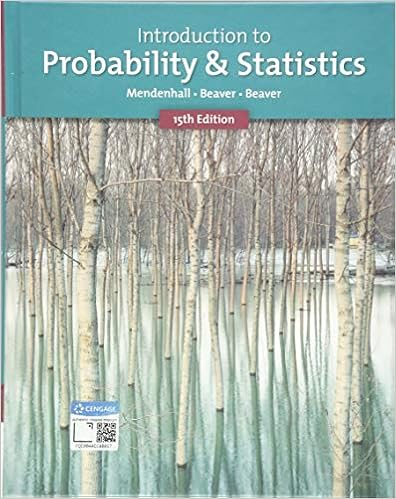# Could this data have been analyzed with one way

• Test Prep
• 21
• 97% (30) 29 out of 30 people found this document helpful

This preview shows page 21 out of 21 pages.

##### We have textbook solutions for you!
The document you are viewing contains questions related to this textbook.The document you are viewing contains questions related to this textbook.
Chapter 10 / Exercise 3
Introduction to Probability and Statistics
Beaver/MendenhallExpert Verified
level. Could this data have been analyzed with one-way, independent groups ANOVA? If so, what do you think the value of Fobt would be? Would the result be significant with the ANOVA analysis too? Explain. ANS: Answer not provided. PTS: 1 8. Which is more powerful, a priori or a posteriori comparisons? Why? ANS: Answer not provided. PTS: 1 MSC: WWW 9.Explain how the Q distributions were developed. How does this compare to the t distributions? ANS: Answer not provided. PTS: 1 10. Which test is more powerful, the TukeyHSD test or the Newman-Keuls test? Explain. ANS: Answer not provided. PTS: 1 11. Give two conditions that should be met before doing a priori comparisons that are not independent. ANS: Answer not provided. PTS: 1 12. Is the Type I error rate higher when doing a priori comparisons or when doing the Tukey HSD test? Explain. ANS: Answer not provided. PTS: 1 13. Write the number of the symbol shown in Column 1 in the space provided to the left of the term in Column 2 that it represents. Column 1 Symbols Column 2Terms 1. sW2 2. 3. sB2 4. SSW 5. 6. SSB ___ Between-groups sum of squares ___ with-in groups sum of squares ___ indicates the mathematical operations of squaring all scores, then summing the squared values ___ between-groups variance estimate ___ within-groups variance estimate ANS: Answer not provided. PTS: 1 MSC: WWW
##### We have textbook solutions for you!
The document you are viewing contains questions related to this textbook.The document you are viewing contains questions related to this textbook.
Chapter 10 / Exercise 3
Introduction to Probability and Statistics
Beaver/MendenhallExpert Verified
•••